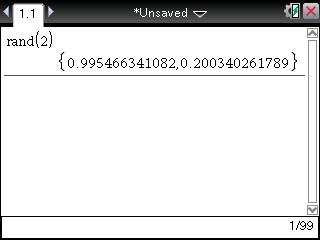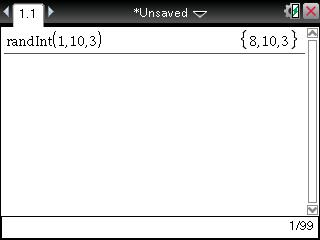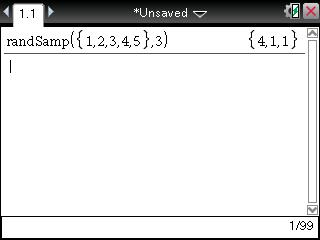# Knowledge Base

## Solution 32271: Creating Random Integers and Numbers Using the TI-Nspire™ Family.

### How do I create random numbers and integers using the TI-Nspire family line of products.

The TI-Nspire family has multiple options for creating random numbers and integers.

For all examples, see the steps below:

First, press [home] [A] or select to add a Calculator page.

To find two random decimal numbers between 0 and 1:

1) Press [menu]    or [R] [A] [N] [D] [(].
2) Press  [enter].To find three random integers between two given values of one and ten:

1) Press [menu]    or [R] [A] [N] [D] [I] [N] [T] [(].
2) Press  [,]   [,]  [enter].To find three numbers chosen at random from a specified list of values of one through five:

1) Press [menu]    or [R] [A] [N] [D] [S] [A] [M] [P] [(].
2) Press [ctrl] [{ }].
3) Press  [,]  [,]  [,]  [,] .
4) Press [tab] [,]  [enter].The seed is the value that is used to determine what random numbers are created. The default seed of the calculator is zero.

To reset the seed that is used to calculate random numbers:

1) Press [menu]   .
2) Press  [enter].